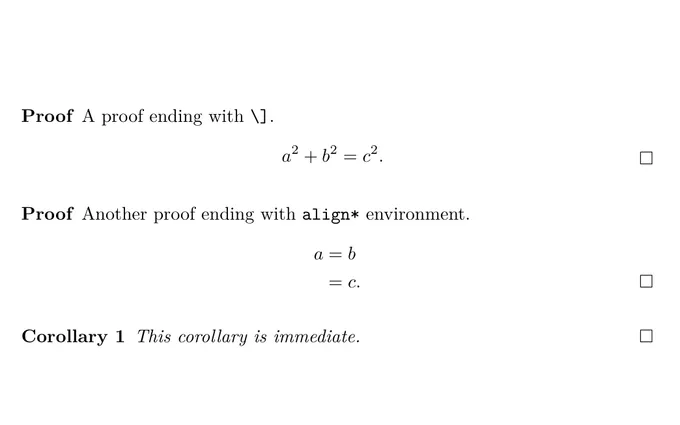# [原创] LaTeX 中优雅地使用证明结束符\documentclass{article}

\usepackage{amssymb}
\usepackage[tbtags]{amsmath}
\usepackage[thmmarks,amsmath]{ntheorem} %%放在 amsmath 之后

\qedsymbol{\ensuremath{\square}} %%在如无需证明的推论等定理类环境中使用 \qed 以显示证明结束符, 此处使用 \ensuremath{\square} 而不是 $\square$ 以便在以数学环境结束的证明中也可以正常使用.
{   % 利用分组，格式设置只作用于证明环境  不出现证明结束符查看ntheorem的thmmarks参数加了没
\theoremstyle{nonumberplain}
\theorembodyfont{\normalfont}
\theoremsymbol{\ensuremath{\square}} %%\square 需要 amssymb 的支持
\newtheorem{proof}{Proof}
}

\newtheorem{corollary}{Corollary}

\begin{document}

\begin{proof}
A proof ending with \verb|\]|.
$a^2 + b^2 = c^2.$
\end{proof}

\begin{proof}
Another proof ending with \verb|align*| environment.
\begin{align*}
a &= b \\
&= c.
\end{align*}
\end{proof}

\begin{corollary}
This corollary is immediate. \qed %%无需证明的推论结尾时加上 \qed 也可以出现证明结束符, 这个是由 \qedsymbol{\ensuremath{\square}} 定义的
\end{corollary}

\end{document}

\theoremstyle{nonumberplain}